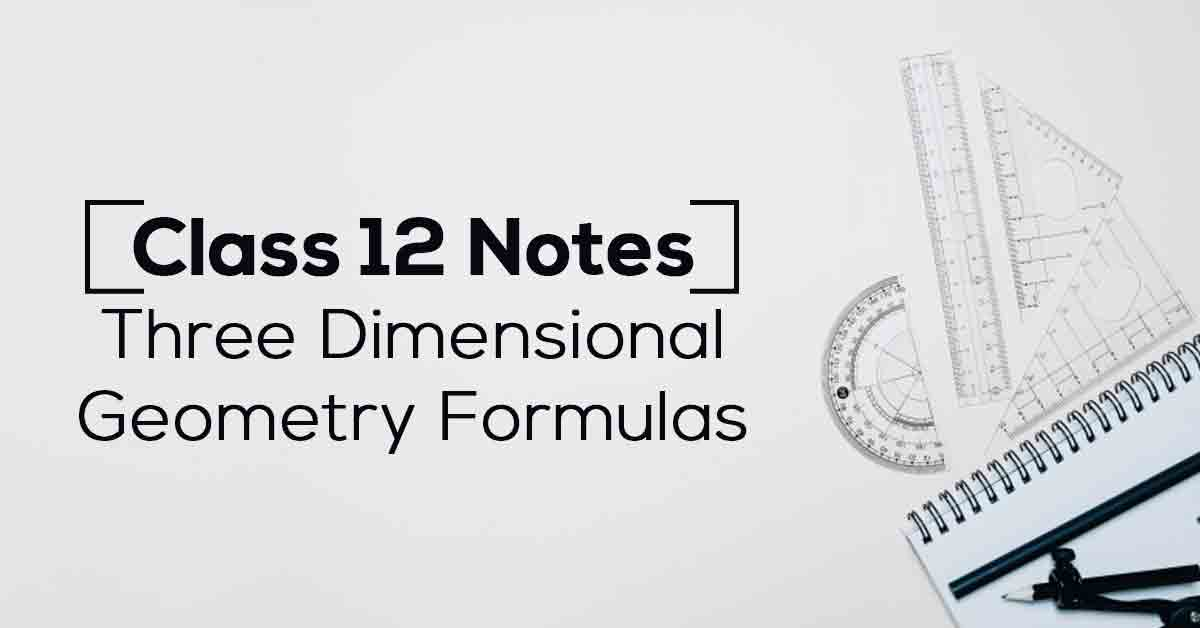Three Dimensional Geometry Class 12 Notes | Vidyakul
×

# Three Dimensional Geometry Class 12 Notes## Class 12 Maths Chapter 11 Three Dimensional Geometry Formulas- PDF Download

In this chapter, we present a vector–algebra approach to three–dimensional geometry. The aim is to present standard properties of lines and planes, with minimum use of complicated three–dimensional diagrams such as those involving similar triangles. Know More about these in Three Dimensional Geometry Class 12 Notes List.

The topics and sub-topics covered in Three Dimensional Geometry Class 12 Notes are:

11.1 Introduction

11.2 Direction Cosines and Direction Ratios of a Line

11.2.1 Relation between the direction cosines of a line

11.2.2 Direction cosines of a line passing through two points

11.3 Equation of a Line in Space

11.3.1 Equation of a line through a given point and parallel to a given vector

11.3.2 Equation of a line passing through two given points

11.4 Angle between Two Lines

11.5 Shortest Distance between Two Lines

11.5.1 Distance between two skew lines

11.5.2 Distance between parallel lines

11.6 Plane

11.6.1 Equation of a plane in normal form

11.6.2 Equation of a plane perpendicular to a given vector and passing through a given point

11.6.3 Equation of a plane passing through three non-collinear points

11.6.4 Intercept form of the equation of a plane

11.6.5 Plane passing through the intersection of two given planes

11.7 Coplanarity of Two Lines

11.8 Angle between Two Planes

11.9 Distance of a Point from a Plane

11.10 Angle between a Line and a Plane.

Download the FREE PDF of Three Dimensional Geometry Class 12 Notes and start your preparation with Vidyakul!